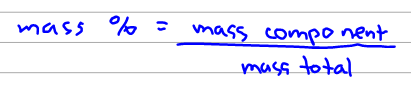# Problem: Calculate the percentage by mass of oxygen in the following compounds.codeine, C18H21NO3

###### FREE Expert Solution

The mass percent can be calculated using the formula below:Before we can solve the mass %, we have to calculate mass of codeine and mass of oxygen.

83% (313 ratings)###### Problem Details

Calculate the percentage by mass of oxygen in the following compounds.

codeine, C18H21NO3